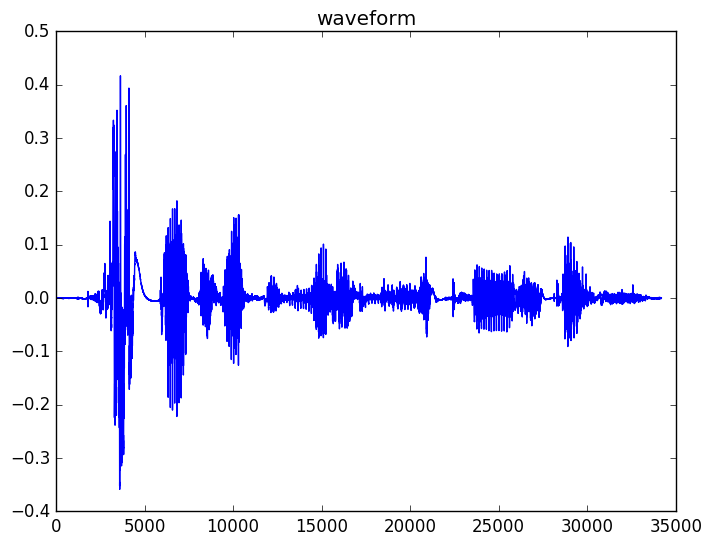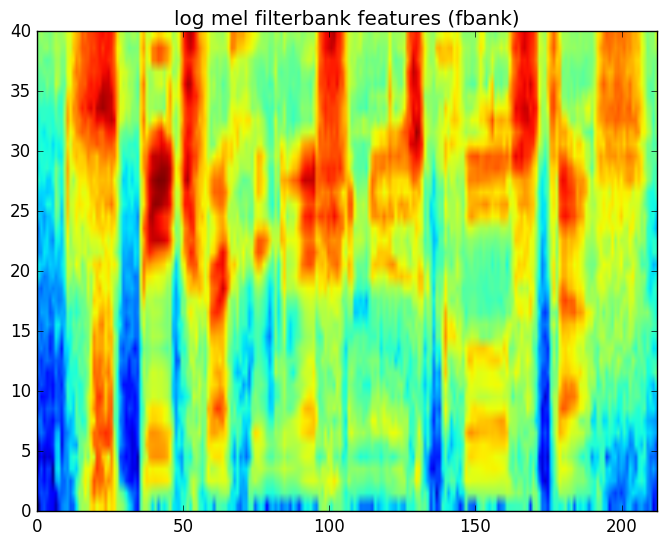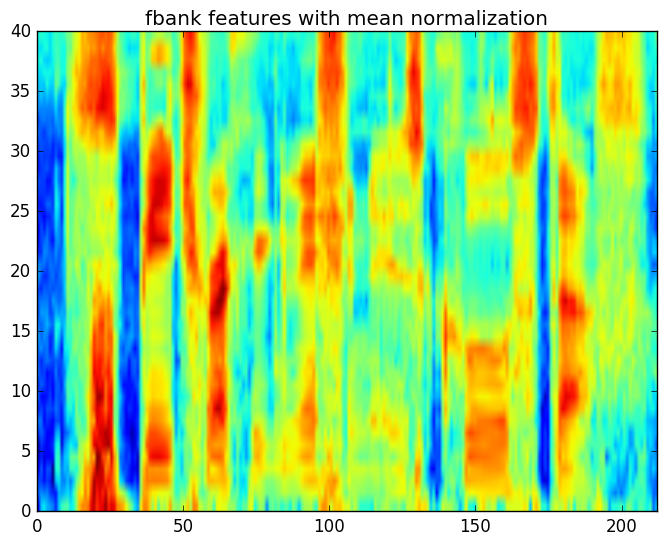# 语音信号处理

Posted by lili on

## Introduction

### Background图：句子”speech recognition is cool stuff”的波形

## 特征提取——短时傅里叶分析

$x_m[n] = w[n] x[mN+n], n=0, 1, \ldots, L-1$

$X_m[k]=\sum_{n=0}^{N-1}x_m[n]e^{-j 2 \pi k n/N}$

$\begin{split} X_m[k+N] & =\sum_{n=0}^{N-1}x_m[n]e^{-j 2 \pi (k+N)n/N} \\ & =\sum_{n=0}^{N-1}x_m[n]e^{-j 2 \pi kn/N}e^{-j2\pi n} \\ & =\sum_{n=0}^{N-1}x_m[n]e^{-j 2 \pi kn/N}=X_m[k] \end{split}$

$\begin{split} X_m[N-k] & = \sum_{n=0}^{N-1}x_m[n]e^{-j 2 \pi (N-k)n/N} \\ & = \sum_{n=0}^{N-1}x_m[n] (cos(2\pi (N-k)n/N)-jsin(2\pi (N-k)n/N)) \\ & = \sum_{n=0}^{N-1}x_m[n] (cos(2\pi kn/N) +j sin(2\pi kn/N) ) \end{split}$

$\begin{split} X_m[k] & = \sum_{n=0}^{N-1}x_m[n]e^{-j 2 \pi kn/N} \\ & = \sum_{n=0}^{N-1}x_m[n] (cos(2\pi kn/N) -j sin(2\pi kn/N) ) \end{split}$

## Mel filtering

$X_{\tt{mel}}[p] = \sum_k M[p,k] \left|X_m[k]\right|$

## Log压缩图：取log后的美尔滤波器特征

## 其它处理

• Dithering
• 增加很小的随机噪声，这样可以防止特征计算的数学问题(比如对零取log)，因为加了随机噪声后频率的每一个部分都有值。
• DC-removal
• 去除直流分量(也就是使得信号的均值为零)
• Pre-emphasis
• 提升原始信号的高频部分。因为语音在低频部分的能量较大和高频较小，这样高低频的能量差别太多，通过下面的公式可以实现提升高频部分的能量。
$y[n] = x[n] - \alpha x[n-1]$

## feature normalization

$X_{t,{\tt obs}}[k] = H[k] X_t[k]$

$\left|X_{t,{\tt obs}}[k]\right| = \left|H[k]\right|\left|X_t[k]\right|$

$\begin{split} \mu_{\tt obs} & =\frac{1}{T}\sum_t \log\left(\left|X_{t,{\tt obs}}[k]\right|\right) \\ & =\frac{1}{T}\sum_t \log\left(\left|H[k]\right|\left|X_t[k]\right|\right) \\ & =\frac{1}{T}\sum_t \log\left(\left|H[k]\right|\right)+\frac{1}{T}\sum_t \log\left(\left|X_t[k]\right|\right) \end{split}$

$\mu_{tt obs}=\log\left(\left|H[k]\right|\right)$图：进行均值归一化之后的fbank特征

## lab2

### Instructions

• Pre-emphasis
• 把语音信号切分成有重叠部分的帧
• 对于每一帧：
• 加窗
• 就是这一帧的幅度谱(magnitude spectrum)
• 对幅度谱使用美尔滤波器组滤波得到FBANK系数
• 对FBANK系数取log

• 波形图
• 美尔滤波器组
• Log的FBANK系数

$python M2_Wav2Feat_Batch.py –set train$ python M2_Wav2Feat_Batch.py –set dev
$python M2_Wav2Feat_Batch.py –set test  对于训练集，除了提取特征之外还会生成feat_mean.ascii和feat_invstddev.ascii，分别表示训练数据的特征的均值和方差(方差的逆)，这些值在后面的声学模型训练时需要用到。 ### 代码阅读和参考实现 请读者一定要自己想办法实现上面的代码，如果实在完成不了再参考本节内容，否则如果直接就看参考代码不会有太大收获。完整代码在这里 #### __init__函数 def __init__(self, samp_rate=16000, frame_duration=0.025, frame_shift=0.010, preemphasis=0.97, num_mel=40, lo_freq=0, hi_freq=None, mean_norm_feat=True, mean_norm_wav=True, compute_stats=False): self.samp_rate = samp_rate self.win_size = int(np.floor(frame_duration * samp_rate)) self.win_shift = int(np.floor(frame_shift * samp_rate)) self.lo_freq = lo_freq if (hi_freq == None): self.hi_freq = samp_rate//2 else: self.hi_freq = hi_freq self.preemphasis = preemphasis self.num_mel = num_mel self.fft_size = 2 while (self.fft_size<self.win_size): self.fft_size *= 2 self.hamwin = np.hamming(self.win_size) self.make_mel_filterbank() self.mean_normalize = mean_norm_feat self.zero_mean_wav = mean_norm_wav self.global_mean = np.zeros([num_mel]) self.global_var = np.zeros([num_mel]) self.global_frames = 0 self.compute_global_stats = compute_stats  这个函数是FrontEnd类的构造函数，主要传入提取特征的一些参数： • samp_rate 采样率，默认16000 • frame_duration 帧长，默认0.025s，也就是25ms • frame_shift 帧移，默认0.010s，也就是10ms • preemphasis preemphasis系数，默认0.97 • num_mel FBANK滤波器组的滤波器个数，默认40 • lo_freq 频率的最小值，默认0 • hi_freq 频率的最大值，默认None，如果None则使用Nyquist频率(sample_rate的一半) • mean_norm_feat 是否对特征进行均值归一化，默认True • mean_norm_wav 是否对特征进行方差归一化，默认True • compute_stats 是否统计所有数据的均值和方程，默认False 代码比较简单，大部分都是把参数保存到self里，下面的代码是计算合适的FFT的点数：  self.fft_size = 2 while (self.fft_size<self.win_size): self.fft_size *= 2  而比较复杂的是make_mel_filterbank()函数，它用来构造滤波器组的，我们来看一下。 def make_mel_filterbank(self): # 把lo_freq和hi_freq从频率变成mel lo_mel = self.lin2mel(self.lo_freq) # 8000->2840 hi_mel = self.lin2mel(self.hi_freq) # uniform spacing on mel scale # 第一个三角形滤波器需要3个点，以后每增加一个滤波器增加一个点 # 因此需要num_mel+2个点，这些点在mel尺度上均匀分布 mel_freqs = np.linspace(lo_mel, hi_mel,self.num_mel+2) # convert mel freqs to hertz and then to fft bins # 采样率是16000，而FFT的点数是512，那么每个点对应的带宽是16000/512=31.25 # typically 31.25 Hz, bin=0 Hz, bin=31.25 Hz,..., bin=8000 Hz bin_width = self.samp_rate/self.fft_size # 把均匀分布的mel_freqs再变成频率，则它们就不均匀了，高频的宽 # 然后除以bin_width则变成fft的id mel_bins = np.floor(self.mel2lin(mel_freqs)/bin_width) num_bins = self.fft_size//2 + 1 self.mel_filterbank = np.zeros([self.num_mel,num_bins]) for i in range(0,self.num_mel): # 三角形滤波器的第一个点的fft的idx left_bin = int(mel_bins[i]) # 第二个点的idx center_bin = int(mel_bins[i+1]) # 第三个点的idx right_bin = int(mel_bins[i+2]) # 第一个点和第二个点形成往上走的直线 up_slope = 1/(center_bin-left_bin) for j in range(left_bin,center_bin): self.mel_filterbank[i,j] = (j - left_bin)*up_slope # 第二个点和第三个点是往下走的直线 down_slope = -1/(right_bin-center_bin) for j in range(center_bin,right_bin): self.mel_filterbank[i,j] = (j-right_bin)*down_slope  频率变成mel和mel变成频率的函数为：  # linear-scale frequency (Hz) to mel-scale frequency def lin2mel(self,freq): return 2595*np.log10(1+freq/700) # mel-scale frequency to linear-scale frequency def mel2lin(self,mel): return (10**(mel/2595)-1)*700  ### process_utterance 这个函数的输入utterance是一个一维的numpy数组，每一个值都是浮点数。  def process_utterance(self, utterance): wav = self.dither(utterance) wav = self.pre_emphasize(wav) frames = self.wav_to_frames(wav) magspec = self.frames_to_magspec(frames) fbank = self.magspec_to_fbank(magspec) if (self.mean_normalize): fbank = self.mean_norm_fbank(fbank) if (self.compute_global_stats): self.accumulate_stats(fbank) return fbank  它会对utterance进行如下的处理： • dither函数增加随机的噪声 • pre_emphasize • wav_to_frames实现分帧 • frames_to_magspec FFT并且计算幅度谱 • magspec_to_fbank 计算log的FBANK系数 • mean_norm_fbank 对log的FBANK系数进行归一化 • accumulate_stats 计算全局的统计信息 ### dither  def dither(self, wav): n = 2*np.random.rand(wav.shape)-1 n *= 1/(2**15) return wav + n  增加很小的随机噪声。 ### pre_emphasize  def pre_emphasize(self, wav): # apply pre-emphasis filtering on waveform preemph_wav = [] preemph_wav.append(wav) for i in range(1, len(wav)): preemph_wav.append(wav[i] - self.preemphasis * wav[i - 1]) return np.asarray(preemph_wav)  使用前面的公式$y[n] = x[n] - \alpha x[n-1]$计算。 ### wav_to_frames  def wav_to_frames(self, wav): # only process whole frames num_frames = int(np.floor((wav.shape - self.win_size) / self.win_shift) + 1) frames = np.zeros([self.win_size, num_frames]) for t in range(0, num_frames): frame = wav[t * self.win_shift:t * self.win_shift + self.win_size] if (self.zero_mean_wav): frame = frame - np.mean(frame) frames[:, t] = self.hamwin * frame return frames  wav_to_frames实现分帧，首先计算帧数：帧数=(wav样本数-帧长)/帧移+1。然后就是简单的从wav中截取对于的数据，最后乘以窗函数self.hamwin。 ### frames_to_magspec  def frames_to_magspec(self, frames): complex_spec = np.fft.rfft(frames, self.fft_size, 0) magspec = np.absolute(complex_spec) return magspec  使用np.fft.rfft计算FFT，这里使用rfft表示是对实数进行FFT，它返回的点数为self.fft_size/2+1。然后用np.absolute对复数求其幅度(模)。 ### magspec_to_fbank  def magspec_to_fbank(self, magspec): fbank = np.dot(self.mel_filterbank, magspec) return np.log(fbank)  计算fbank系数，就是把mel_filterbank和magspec相乘。最后把系数取log。 ### mean_norm_fbank  def mean_norm_fbank(self, fbank): # compute mean fbank vector of all frames # subtract it from each frame mean=np.mean(fbank, axis=1).reshape(-1,1) return fbank-mean  对fbank的系数的每一维进行均值归一化(使得均值为零) ### accumulate_stats  def accumulate_stats(self, fbank): self.global_mean += np.sum(fbank,axis=1) self.global_var += np.sum(fbank**2,axis=1) self.global_frames += fbank.shape  统计全局的均值、方差，用于生成feat_mean.ascii和feat_invstddev.ascii。 ### M2_Wav2Feat_Single.py 我们简单的来看一下这个脚本，它的作用是提取训练、开发和测试集的特征。 下面以训练集为例。它的输入是Experiments/lists/wav_train.list，我们来看一下： $ head wav_train.list
LibriSpeech/dev-clean/1272/128104/1272-128104-0000.flac
LibriSpeech/dev-clean/1272/128104/1272-128104-0001.flac
LibriSpeech/dev-clean/1272/128104/1272-128104-0002.flac
LibriSpeech/dev-clean/1272/128104/1272-128104-0003.flac
LibriSpeech/dev-clean/1272/128104/1272-128104-0005.flac
LibriSpeech/dev-clean/1272/128104/1272-128104-0006.flac
LibriSpeech/dev-clean/1272/128104/1272-128104-0007.flac
LibriSpeech/dev-clean/1272/128104/1272-128104-0008.flac
LibriSpeech/dev-clean/1272/128104/1272-128104-0009.flac
LibriSpeech/dev-clean/1272/128104/1272-128104-0010.flac



\$ head feat_train.rscp
1272-128104-0000.feat=.../../feat/1272-128104-0000.feat[0,583]
1272-128104-0001.feat=.../../feat/1272-128104-0001.feat[0,479]
1272-128104-0002.feat=.../../feat/1272-128104-0002.feat[0,1246]
1272-128104-0003.feat=.../../feat/1272-128104-0003.feat[0,987]
1272-128104-0005.feat=.../../feat/1272-128104-0005.feat[0,898]
1272-128104-0006.feat=.../../feat/1272-128104-0006.feat[0,561]
1272-128104-0007.feat=.../../feat/1272-128104-0007.feat[0,921]
1272-128104-0008.feat=.../../feat/1272-128104-0008.feat[0,509]
1272-128104-0009.feat=.../../feat/1272-128104-0009.feat[0,1826]
1272-128104-0010.feat=.../../feat/1272-128104-0010.feat[0,557]


    samp_rate = 16000
fe = sp.FrontEnd(samp_rate=samp_rate, mean_norm_feat=True, compute_stats=compute_stats)

for line in wav_files:

feat = fe.process_utterance(x)
# 输出特征文件
htk.write_htk_user_feat(feat, feat_file)
feat_rscp_line = os.path.join(rscp_dir, '..', 'feat', feat_name)
# 在rscp里输出一行，类似1272-128104-0000.feat=.../../feat/1272-128104-0000.feat[0,583]
out_list.write("%s=%s[0,%d]\n" % (feat_name, feat_rscp_line,feat.shape-1))
count += 1

if (compute_stats):
m, p = fe.compute_stats() # m=mean, p=precision (inverse standard deviation)
htk.write_ascii_stats(m, mean_file)
print("Wrote global mean to", mean_file)
htk.write_ascii_stats(p, invstddev_file)
print("Word global inv stddev to ", invstddev_file)